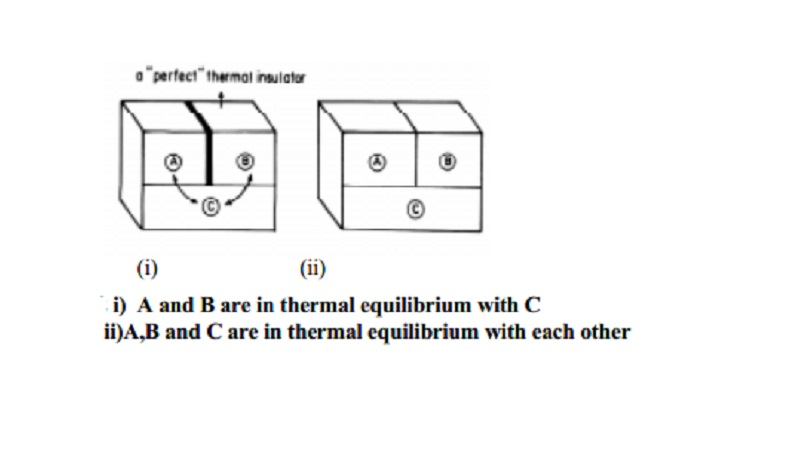Home | | Chemistry | | Chemistry | Zeroth law of thermodynamics or Thermal equilibrium

# Zeroth law of thermodynamics or Thermal equilibriumZeroth law of thermodynamics is also known as the law of thermal equilibrium. It provides a logical basis for the concept of temperature of a system. It can be stated as follows.

Zeroth law of thermodynamics

Consider any two objects each maintained at different temperature, when brought in thermal contact with each other such that heat is exchanged until a thermal equilibrium is reached, then the two objects are considered to have equal temperatures. For example, if a beaker containing water and a thermometer are the two objects, while reading the temperature of the water in the beaker using the thermometer, a thermal equilibrium is reached between the two objects having a contact with each other. Also, when the temperatures of the thermometer bulb and that of water in the beaker are same, thermal equilibrium has said to be occurred.

Zeroth law of thermodynamics is also known as the law of thermal equilibrium. It provides a logical basis for the concept of temperature of a system. It can be stated as follows.

`If two systems at different temperatures are separately in thermal equilibrium with a third one, then they tend to be in thermal equilibrium with themselves'.

Conversely, the Zeroth law can be stated in another manner as,

`When two objects are in thermal equilibrium with the third object, then there is thermal equilibrium between the two objects itself'.

Study Material, Lecturing Notes, Assignment, Reference, Wiki description explanation, brief detail

Related Topics